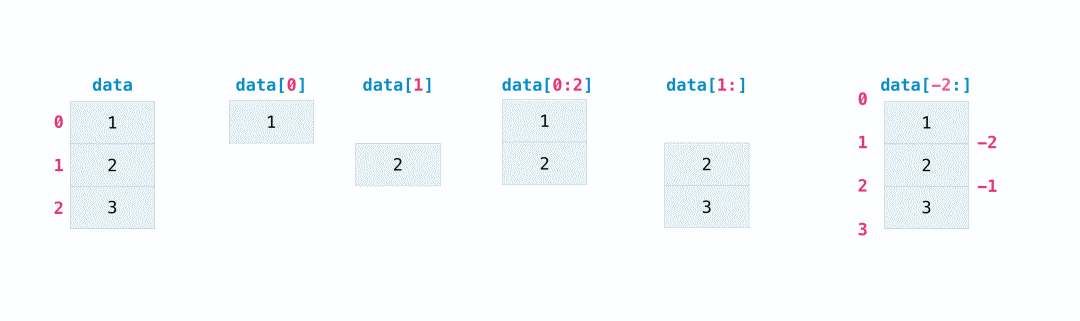NumPy索引与切片的用法示例总结

索引和切片

>>> data = np.array([1, 2, 3])

>>> data
2
>>> data[0:2]
array([1, 2])
>>> data[1:]
array([2, 3])
>>> data[-2:]
array([2, 3])>>> a = np.array([[1 , 2, 3, 4], [5, 6, 7, 8], [9, 10, 11, 12]])

>>> print(a[a < 5])
[1 2 3 4]

>>> five_up = (a >= 5)
>>> print(a[five_up])
[ 5  6  7  8  9 10 11 12]

>>> divisible_by_2 = a[a%2==0]
>>> print(divisible_by_2)
[ 2  4  6  8 10 12]

>>> c = a[(a > 2) & (a < 11)]
>>> print(c)
[ 3  4  5  6  7  8  9 10]

>>> five_up = (a > 5) | (a == 5)
>>> print(five_up)
[[False False False False]
[ True  True  True  True]
[ True  True  True True]]

>>> a = np.array([[1, 2, 3, 4], [5, 6, 7, 8], [9, 10, 11, 12]])

>>> b = np.nonzero(a < 5)
>>> print(b)
(array([0, 0, 0, 0]), array([0, 1, 2, 3]))

>>> list_of_coordinates= list(zip(b, b))

>>> for coord in list_of_coordinates:
...     print(coord)
(0, 0)
(0, 1)
(0, 2)
(0, 3)

>>> print(a[b])
[1 2 3 4]

>>> not_there = np.nonzero(a == 42)
>>> print(not_there)
(array([], dtype=int64), array([], dtype=int64))

总结